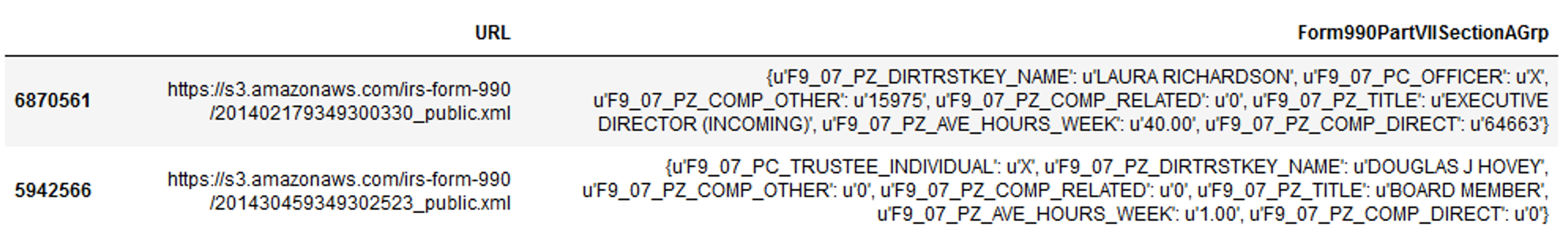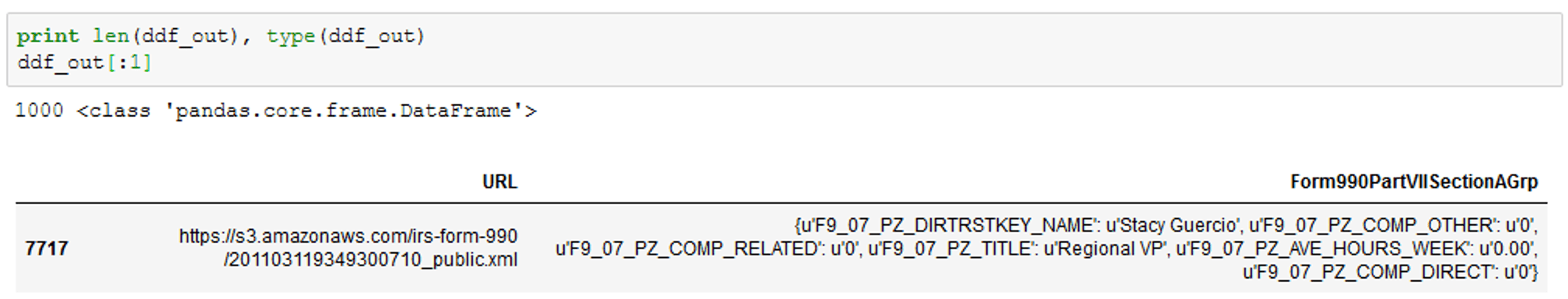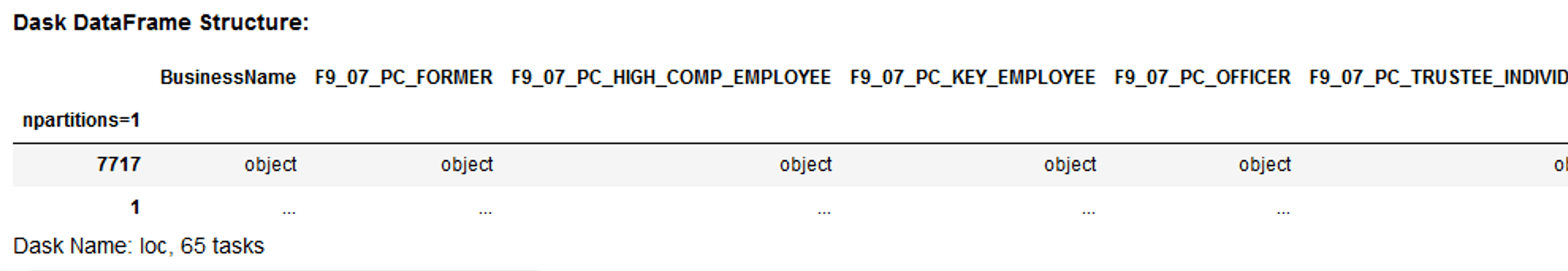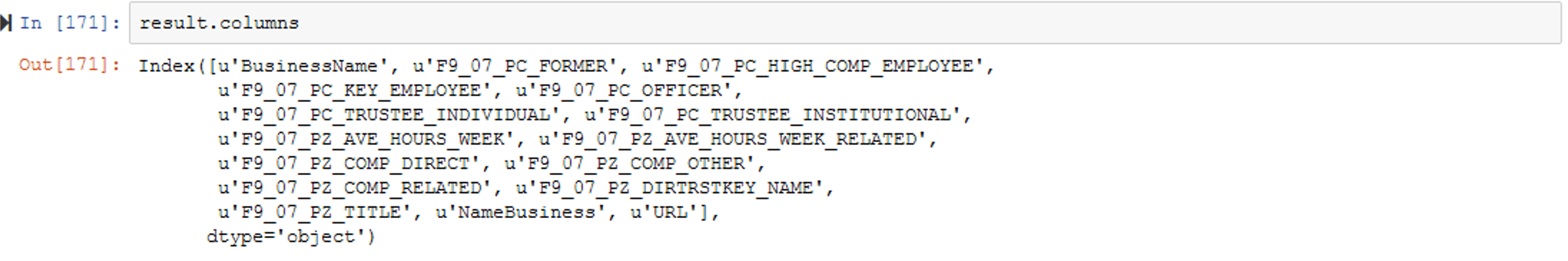# #StackBounty: #python #pandas #dask #flatten Dask to Flatten Dictionary Column

### Bounty: 50

I am new to Dask and am looking to find a way to flatten a dictionary column in a PANDAS dataframe. Here is a screenshot of the first row of a 16 million-row dataframe:I would normally flatten the Form990PartVIISectionAGrp column with the following code:

``````    df = pd.concat([df.drop(['Form990PartVIISectionAGrp'], axis=1), df['Form990PartVIISectionAGrp'].swifter.apply(pd.Series)], axis=1)
``````

I’m looking to do this in Dask but am getting the following error: “ValueError: The columns in the computed data do not match the columns in the provided metadata.”

I am using Python 2.7. I import the relevant packages

``````    from dask import dataframe as dd
from dask.multiprocessing import get
from multiprocessing import cpu_count
nCores = cpu_count()
``````

To test the code, I created a random sample of the data:

``````    dfs = df.sample(1000)
``````

And then generate the Dask dataframe:

``````    ddf = dd.from_pandas(dfs, npartitions=nCores)
``````

The column is currently in string format so I convert it to a dictionary. Normally, I would just write one line of code:

``````dfs['Form990PartVIISectionAGrp'] = dfs['Form990PartVIISectionAGrp'].apply(literal_eval)
``````

But I’ve instead tried to do it here in a more ‘Dask-like’ form so I write the following function and then apply it:

``````    def make_dict(dfs):
dfs['Form990PartVIISectionAGrp'] = dfs['Form990PartVIISectionAGrp'].apply(literal_eval)
return dfs
``````
``````    ddf_out = ddf.map_partitions(make_dict, meta=dfs[:0]).compute()
``````

This works — it returns a PANDAS dataframe where the Form990PartVIISectionAGrp column is in dictionary format (it’s not any faster than the non-Dask apply, however).I then re-create the Dask DF:

``````    ddf = dd.from_pandas(ddf_out, npartitions=nCores)
``````

And write a function to flatten the column:

``````    def flatten(ddf_out):
ddf_out = pd.concat([ddf_out.drop(['Form990PartVIISectionAGrp'], axis=1), ddf_out['Form990PartVIISectionAGrp'].apply(pd.Series)], axis=1)
#ddf_out = ddf_out['Form990PartVIISectionAGrp'].apply(pd.Series)
return ddf_out
``````

If I then run this code:

``````    result = ddf.map_partitions(flatten)
``````

I get the following output, where the column has not been flattened:I was also getting errors about missing meta data, and given that the above did not help parse the dictionary column, so I created a list of the columns produced by the plain Python flattening of the columns and used that to create a dictionary of the columns and data types:

``````metadir = {u'BusinessName': 'O', u'F9_07_PC_FORMER': 'O', u'F9_07_PC_HIGH_COMP_EMPLOYEE': 'O',
u'F9_07_PC_KEY_EMPLOYEE': 'O', u'F9_07_PC_OFFICER': 'O',
u'F9_07_PC_TRUSTEE_INDIVIDUAL': 'O', u'F9_07_PC_TRUSTEE_INSTITUTIONAL': 'O',
u'F9_07_PZ_AVE_HOURS_WEEK': 'O', u'F9_07_PZ_AVE_HOURS_WEEK_RELATED': 'O',
u'F9_07_PZ_COMP_DIRECT': 'O', u'F9_07_PZ_COMP_OTHER': 'O',
u'F9_07_PZ_COMP_RELATED': 'O', u'F9_07_PZ_DIRTRSTKEY_NAME': 'O',
u'F9_07_PZ_TITLE': 'O', u'NameBusiness': 'O', u'URL': 'O'}
``````

I then apply the flatten function with this meta data:

``````    result = ddf.map_partitions(flatten, meta=metadir)
``````

I get the following output as result:Running result.columns yields this:Where this fails is in running compute(), where I get the following error message: “ValueError: The columns in the computed data do not match the columns in the provided metadata.” I get the same error whether I write:

``````result.compute()
``````

or

``````result.compute(meta=metadir)
``````

I’m not sure what I’m doing wrong here. The columns in result seem to match those in metadir. Any suggestions would be greatly appreciated.

Get this bounty!!!

This site uses Akismet to reduce spam. Learn how your comment data is processed.